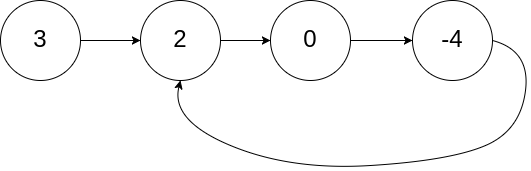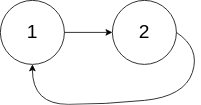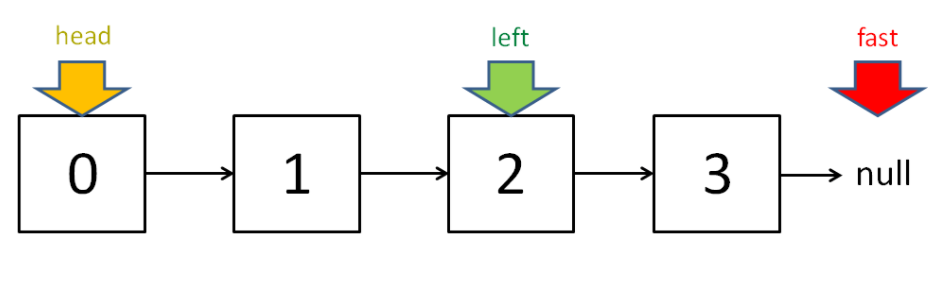1. 双指针

# 双指针

## 快慢指针

### 141-判定链表中是否含有环``````输入：head = [3,2,0,-4], pos = 1

````````````输入：head = [1,2], pos = 0

````````````输入：head = , pos = -1

``````

• 链表中节点的数目范围是 `[0, 104]`
• `-105 <= Node.val <= 105`
• `pos``-1` 或者链表中的一个 有效索引

``````boolean hasCycle(ListNode head) {
return false;
}
``````

• 如果不含有环，跑得快的那个指针最终会遇到 `null`，说明链表不含环；
• 如果含有环，快指针最终会超慢指针一圈，和慢指针相遇，说明链表含有环。
``````/**
* class ListNode {
*     int val;
*     ListNode next;
*     ListNode(int x) {
*         val = x;
*         next = null;
*     }
* }
*/
public class Solution {
ListNode fast, slow;
while (fast != null && fast.next != null) {
fast = fast.next.next;
slow = slow.next;

if (fast == slow) {
return true;
}
}

return false;
}
}
``````

### 142-已知链表中含有环，返回这个环的起始位置``````输入：head = [3,2,0,-4], pos = 1

````````````输入：head = [1,2], pos = 0

````````````输入：head = , pos = -1

``````

• 链表中节点的数目范围在范围 `[0, 104]`
• `-105 <= Node.val <= 105`
• `pos` 的值为 `-1` 或者链表中的一个有效索引`fast` 一定比 `slow` 多走了 `k` 步，这多走的 `k` 步其实就是 `fast` 指针在环里转圈圈，所以 `k` 的值就是环长度的「整数倍」。``````/**
* class ListNode {
*     int val;
*     ListNode next;
*     ListNode(int x) {
*         val = x;
*         next = null;
*     }
* }
*/
public class Solution {
ListNode fast, slow;
while (fast != null && fast.next != null) {
fast = fast.next.next;
slow = slow.next;

if (fast == slow) {
break;
}
}

if (fast == null || fast.next == null) {
return null;
}

while (slow != fast) {
slow = slow.next;
fast = fast.next;
}

return slow;
}
}
``````

### 876-链表的中间结点

``````输入：[1,2,3,4,5]

ans.val = 3, ans.next.val = 4, ans.next.next.val = 5, 以及 ans.next.next.next = NULL.
``````

``````输入：[1,2,3,4,5,6]

``````

• 给定链表的结点数介于 `1``100` 之间。``````/**
* public class ListNode {
*     int val;
*     ListNode next;
*     ListNode() {}
*     ListNode(int val) { this.val = val; }
*     ListNode(int val, ListNode next) { this.val = val; this.next = next; }
* }
*/
class Solution {
ListNode slow, fast;
while (fast != null && fast.next != null) {
slow = slow.next;
fast = fast.next.next;
}

// slow 就在中间位置
return slow;
}
}
``````

### 19-删除链表的倒数第N个结点

• 给你一个链表，删除链表的倒数第 `n` 个结点，并且返回链表的头结点。

示例 1：``````输入：head = [1,2,3,4,5], n = 2
输出：[1,2,3,5]
``````

示例 2：

``````输入：head = , n = 1
输出：[]
``````

示例 3：

``````输入：head = [1,2], n = 1
输出：
``````

提示：

• 链表中结点的数目为 `sz`
• `1 <= sz <= 30`
• `0 <= Node.val <= 100`
• `1 <= n <= sz`

``````class Solution {
public ListNode removeNthFromEnd(ListNode head, int n) {
ListNode fast, slow;
// 快指针先前进 n 步
while (n-- > 0) {
fast = fast.next;
}

if (fast == null) {
// 如果此时快指针走到头了，
// 说明倒数第 n 个节点就是第一个节点
}

// 让慢指针和快指针同步向前
while (fast != null && fast.next != null) {
slow = slow.next;
fast = fast.next;
}

// slow.next 就是倒数第 n 个节点，删除它
slow.next = slow.next.next;
}
}
``````

## 左右指针

### 二分查找

``````int binarySearch(int[] nums, int target) {
int left = 0;
int right = nums.length - 1;
while(left <= right) {
int mid = (right + left) / 2;
if(nums[mid] == target)
return mid;
else if (nums[mid] < target)
left = mid + 1;
else if (nums[mid] > target)
right = mid - 1;
}

return -1;
}
``````

### 167-两数之和-输入有序数组

``````输入：numbers = [2,7,11,15], target = 9

``````

``````输入：numbers = [2,3,4], target = 6

``````

``````输入：numbers = [-1,0], target = -1

``````

• `2 <= numbers.length <= 3 * 104`
• `-1000 <= numbers[i] <= 1000`
• `numbers`非递减顺序 排列
• `-1000 <= target <= 1000`
• 仅存在一个有效答案

``````class Solution {
public int[] twoSum(int[] nums, int target) {
int left = 0, right = nums.length-1;
while(left < right) {
int sum = nums[left] + nums[right];
if (sum == target) {
return new int[]{ left+1, right+1 };
} else if (sum < target) {
left++;
} else {
right--;
}
}

return new int[] { -1, -1 };
}
}
``````

### 344-反转字符串

``````输入：s = ["h","e","l","l","o"]

``````

``````输入：s = ["H","a","n","n","a","h"]

``````

• `1 <= s.length <= 105`
• `s[i]` 都是 ASCII 码表中的可打印字符

``````void reverseString(char[] arr) {
int left = 0;
int right = arr.length - 1;
while (left < right) {
// 交换 arr[left] 和 arr[right]
char temp = arr[left];
arr[left] = arr[right];
arr[right] = temp;
left++; right--;
}
}
``````

``````class Solution {
public void reverseString(char[] s) {
int left = 0, right = s.length-1;
while (left < right) {
s[left] ^= s[right];
s[right] ^= s[left];
s[left] ^= s[right];
left++; right--;
}
}
}
``````

×